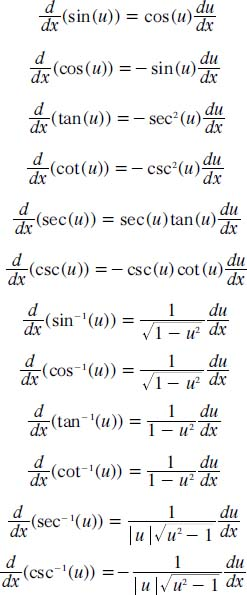NextPrevious

# What are the derivatives of trigonometric functions?

There are also derivatives of the six major trigonometric functions—sine, cosine, cotangent, cosecant, tangent, and secant (for more information on these trigonometric functions, see “Geometry and Trigonometry”). The following lists the formulas for those derivatives (in this case, the functions are written with respect to the variable u):Close

This is a web preview of the "The Handy Math Answer Book" app. Many features only work on your mobile device. If you like what you see, we hope you will consider buying. Get the App